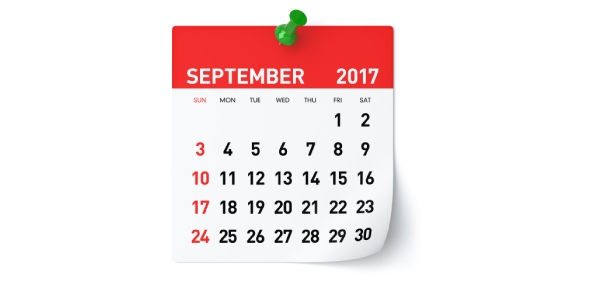# September Assessment

10 Questions | Total Attempts: 21SettingsSeptember Assessment

Related Topics
• 1.
1.       How many core counties are there ?
• A.

12 Major core counties

• B.

13 Major core counties

• C.

14 Major core counties

• D.

15 Major core counties

• 2.
Give the expansion for PIDN .
• A.

Personal identification Number

• B.

Property identification Number

• C.

Parcel identification Number

• D.

Personal property identification Number

• 3.
Give the expansion of DBA.
• A.

• B.

Data base Associate

• C.

Data base Associate consultant

• 4.
How many core couties has been worked by murali’s team till date?
• A.

5 Counties

• B.

6 Counties

• C.

13 Counties

• D.

4 Counties

• E.

3 Counties

• 5.
How may fields are there in the master file ?
• A.

100 fields

• B.

120 fields

• C.

88 fields

• D.

99 fields

• E.

87 fields

• 6.
What is the reason for verifying and updating the Land squarefeet and gross square feet.
• A.

To ensure that data base is correct

• B.

To ensure that the year is correct

• C.

To ensure that the datas in our DB is updated as per the current year status .

• D.

To ensure that the information on CAD site is correct

• 7.
What is square yard in squarefoot?
• A.

One square yard is sixsquare feet.

• B.

One square yard is fivesquare feet.

• C.

One square yard is nine square feet.

• D.

One square yard is twelve square feet.

• E.

One square yard is nine tenfeet.

• 8.
What is acre ?
• A.

Land measurement that is equal to 43,560 square feet or 12/640 square mile

• B.

Land measurement that is equal to 43,560 square feet or 1/540 square mile

• C.

Land measurement that is equal to 43,000 square feet or 1/640 square mile

• D.

Land measurement that is equal to 43,560 square feet or 1/64000 square mile

• E.

Land measurement that is equal to 43,560 square feet or 1/640 square mile

• 9.
How will you convert 1 acre of land to land square feet?
• A.

Land square feet = 1*43560 = 43560 Sqft .

• B.

1 acre = 1*43560 = 43560 Sqft .

• C.

Land square feet = 2*43560 = 43560 Sqft .

• D.

1 acre = 2*43560 = 43560 Sqft .

• 10.
Identify the Gross square feet for the below mentioned house A house that had a main floor of 1999 square feet, a lower floor of 2304 square feet, a basement of usable space of 980 square feet, a garage of 880 square feet and two covered decks of 440 square feet .
• A.

Total Grosssq. ft. of: 6,703 square feet.

• B.

Total Grosssq. ft. of: 6,000 square feet.

• C.

Total Grosssq. ft. of: 6,603 square feet.

• D.

Total Grosssq. ft. of: 6,666 square feet.

• E.

Total Grosssq. ft. of: 6,613 square feet.